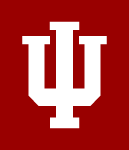Same school, new name. The School of Informatics and Computing is changing its name effective January 11, 2023. Learn more about the name change

# PBHL-B 302 Biostatistics for Informatics

3 credits

• Prerequisites: INFO-I 223
• Delivery: On-Campus
• This course introduces the principles and methods of computational data analysis used in biostatistics, emphasizing examples from public health of sampling, study design, descriptive statistics, probability, statistical distributions, estimation, hypothesis testing, chi-square tests, t-tests, analysis of variance, linear regression, and correlation. Problems in biostatistics are solved by means of programming.

## Learning Outcomes

1. Describe and apply fundamental statistical terms and concepts.
2. Calculate univariate measures of central tendency and variation.
3. Display and interpret data graphically.
4. Test and interpret hypotheses about sample means and goodness of fit.
5. Derive and interpret regression analyses.
6. Generate results by programming in Python.